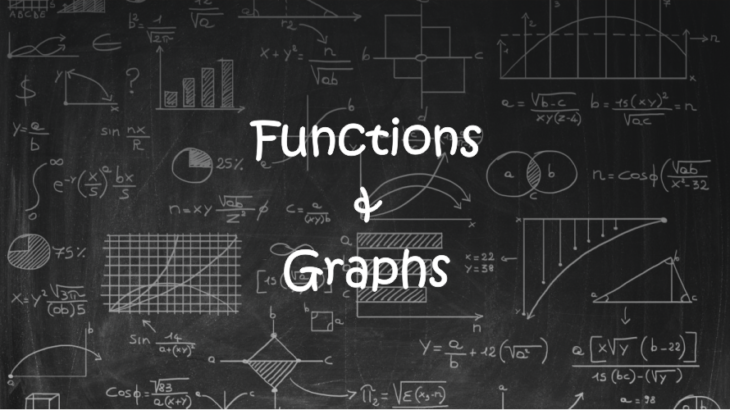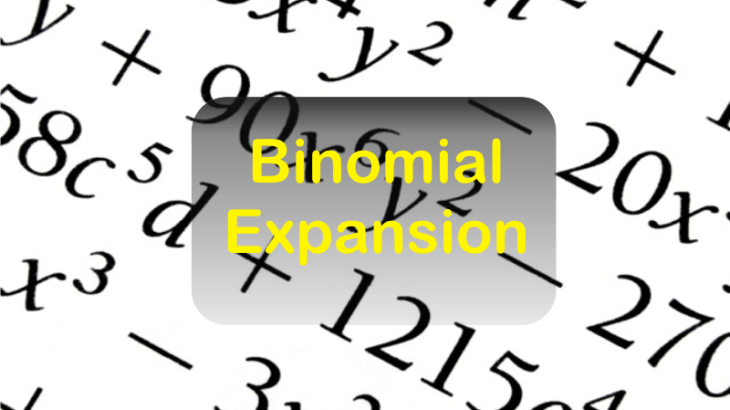## Vocabulary for CAT IIFT SNAP and other MBA Entrance ExamHow important is vocabulary for CAT? Aspirants often wonder as to how essential is the vocabulary for CAT. They must remember that while vocabulary may not directly play a role in fetching your marks, it does help you a great deal in improving your comprehension. The greater your understanding of words the better is your […]

## How to find Main Idea or Central Idea of the RC PassageThe first question in many CAT Reading Comprehension passages will ask you to identify the statement that best expresses the central idea, main idea, or the main point of the passage as a whole. These questions come in different varieties, but in each kind, the question focuses on the central idea or the main theme […]

## [MPG] Mass Point Geometry : Tricks for Triangles for CAT Exam

Introduction to Mass Point Geometry [MPG] Mass point Geometry (MPG) is a technique borrowed from the concepts of physics which we apply to solve many complex problems involving triangles and intersecting lines. The technique is easy to understand and apply. MPG technique also simplifies calculations of ratios in geometric figures in an intuitive way by […]

## Geometry Formulas for Area of the Triangle for CAT Exam

There are many formulas to calculate the area of a triangle. Some formulas are very frequently used and some are used specifically for certain types of questions. A student should not only remember those formulas but also know where to apply them. In this article will discuss all the formulas for finding the area of a […]

## CAT Geometry Concepts and Questions: Triangles

Fundamental concepts of Triangles Under the geometry section of various competitive exams like CAT, the most critical topic within geometry is Triangle. Many questions from triangle are based on the basic concepts like the understanding of sides and angles and a few elementary theorems on triangles. It is interesting to note that the questions can be […]

## Basic Concepts of Functions and Graphs for CAT ExamsIn CAT Exam, certain kind of questions from algebra requires higher levels of algebraic concepts. However, such questions can also be easily solved by using alternate approaches from graphs. Below are a few questions from CAT and other exams, which would be very difficult to crack if we try solving them using the concepts from […]

## NMAT 2018 Exam: Complete details

What is NMAT Exam? NMAT exam is conducted by GMAC. The NMAT scores are accepted by different programs of NMIMS institutes at Mumbai, Bangalore, and Hyderabad. The score is accepted by a few other institutes as well. Eligibility: A candidate with full-time bachelor’s degree in any discipline with a minimum of 50% marks is eligible […]

## Application of Binomial Theorem for Finding Integral SolutionsBinomial Theorem The formula by which any positive integral power of a binomial expression can be expanded in the form of a series is known as Binomial Theorem. (x + y)n = nC0 xn + nC1 xn-1 y + nC2 xn-2 y2+ ……………..+ nCn yn e.g:  (a+b)3= 3C0a3 + 3C1a2b+3C2ab2 + 3C3b3 = a3 + 3a2b + 3ab2 +b3 Note The number of terms in the expansion of (x+ y)n is (n + 1) e. one […]